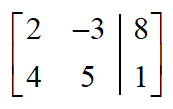# augmented matrix

Augmented Matrix

A matrix form of a linear system of equations obtained from the coefficient matrix as shown below. It is created by adding an additional column for the constants on the right of the equal signs. The new column is set apart by a vertical line.

 System: $$\begin{array}{l}2x - 3y = 8\\4x + 5y = 1\end{array}$$ Augmented Matrix: